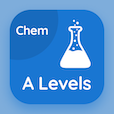Cambridge Online Courses (GCE)

A Level Chemistry MCQs

A Level Chemistry MCQ PDF - Topics

# Introduction to Ionic Equilibria MCQ Quiz Online

Practice Introduction to Ionic Equilibria Multiple Choice Questions (MCQ), Introduction to Ionic Equilibria quiz answers PDF to learn a level chemistry online course for a level chemistry classes. Ionic Equilibria Multiple Choice Questions and Answers (MCQs), Introduction to Ionic Equilibria quiz questions for SAT practice test. "Introduction to Ionic Equilibria MCQ" PDF Book: equilibrium and solubility, buffer solutions, indicators and acid base titrations test prep for colleges that offer online degrees.

"Strong acids completely ionize in" MCQ PDF: introduction to ionic equilibria with choices alcohol, water, gases, and alkalis for SAT practice test. Learn introduction to ionic equilibria quiz questions for merit scholarship test and certificate programs for online college courses.

## MCQs on Introduction to Ionic Equilibria Quiz

MCQ: Strong acids completely ionize in

alcohol
water
gases
alkalis

MCQ: Water may act as

acid only
base only
alkalis
acid and base

MCQ: Water has very low extent of

ionization
bonding
temperature
none of above

MCQ: In compost, the reaction takes place among

weak acids only
weak bases only
strong bases and acids
weak acid and bases

MCQ: The value of ionic product of water is

198K
298K
928K
892K

### More Topics from A Level Chemistry Course